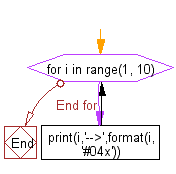﻿ Python Math: Convert an integer to a 2 byte Hex value - w3resource# Python Math: Convert an integer to a 2 byte Hex value

## Python Math: Exercise-80 with Solution

Write a Python program to convert an integer to a 2 byte Hex value.

Sample Solution:-

Python Code:

``````for i in range(1, 10):
print(i,'-->',format(i, '#04x'))
```
```

Sample Output:

```1 --> 0x01
2 --> 0x02
3 --> 0x03
4 --> 0x04
5 --> 0x05
6 --> 0x06
7 --> 0x07
8 --> 0x08
9 --> 0x09
```

Flowchart:## Visualize Python code execution:

The following tool visualize what the computer is doing step-by-step as it executes the said program:

Python Code Editor:

Have another way to solve this solution? Contribute your code (and comments) through Disqus.

What is the difficulty level of this exercise?

Test your Programming skills with w3resource's quiz.

﻿

```>>> students = [{'name': 'John', 'score': 98}, {'name': 'Mike', 'score': 94}, {'name': 'Jennifer', 'score': 99}]